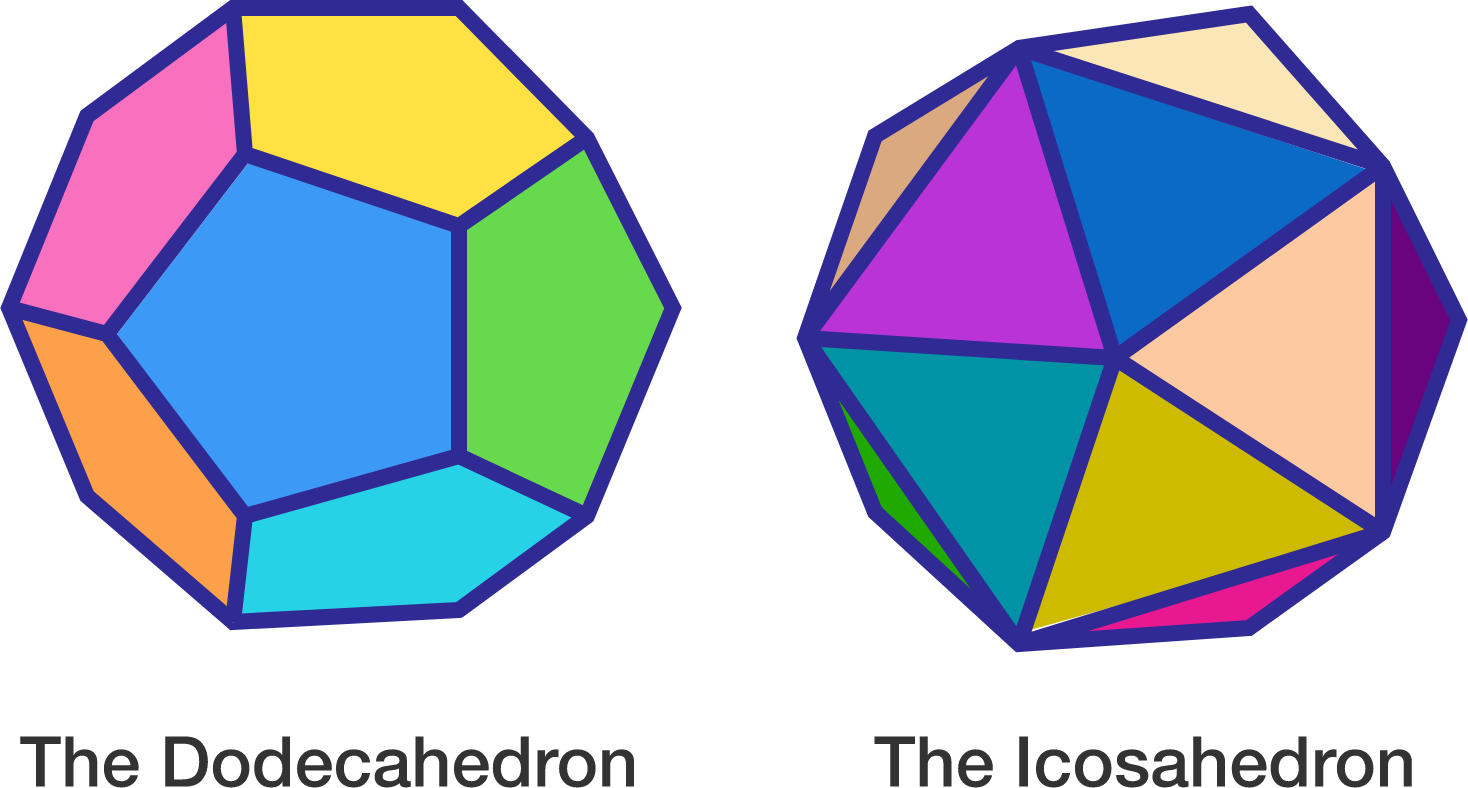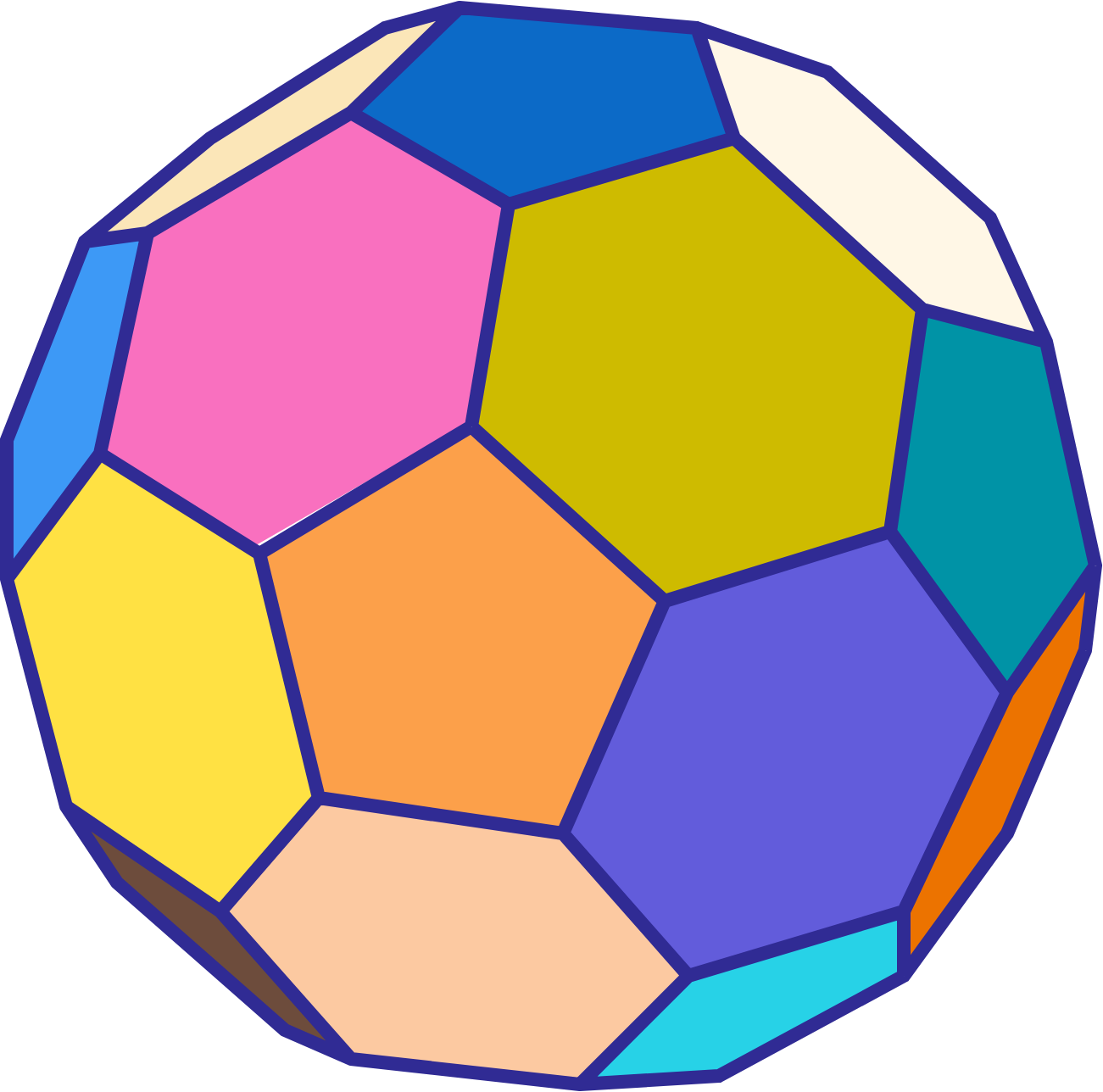# Where do soccer balls come from?

Geometry Level 2The dodecahedron has 12 pentagonal faces. Therefore it has $\frac{12 \times 5}{2} = 30$ edges.

The icosahedron has 20 equilateral triangular faces. Therefore it has $\frac{20 \times 3}{2} = 30$ edges.

How many edges does a truncated icosahedron have?×